Using the IR light source and IR filters we now have a clear separation of signal and noise from our camera. Now we can get to the task of detecting marker positions.

First we'll converting the luminance image into a thresholded binary image. Finally, this image is converted into a a list of marker positions.

# Experiment

Before codifying marker recognition, let's first experiment a bit. We'll be detecting spherical markers: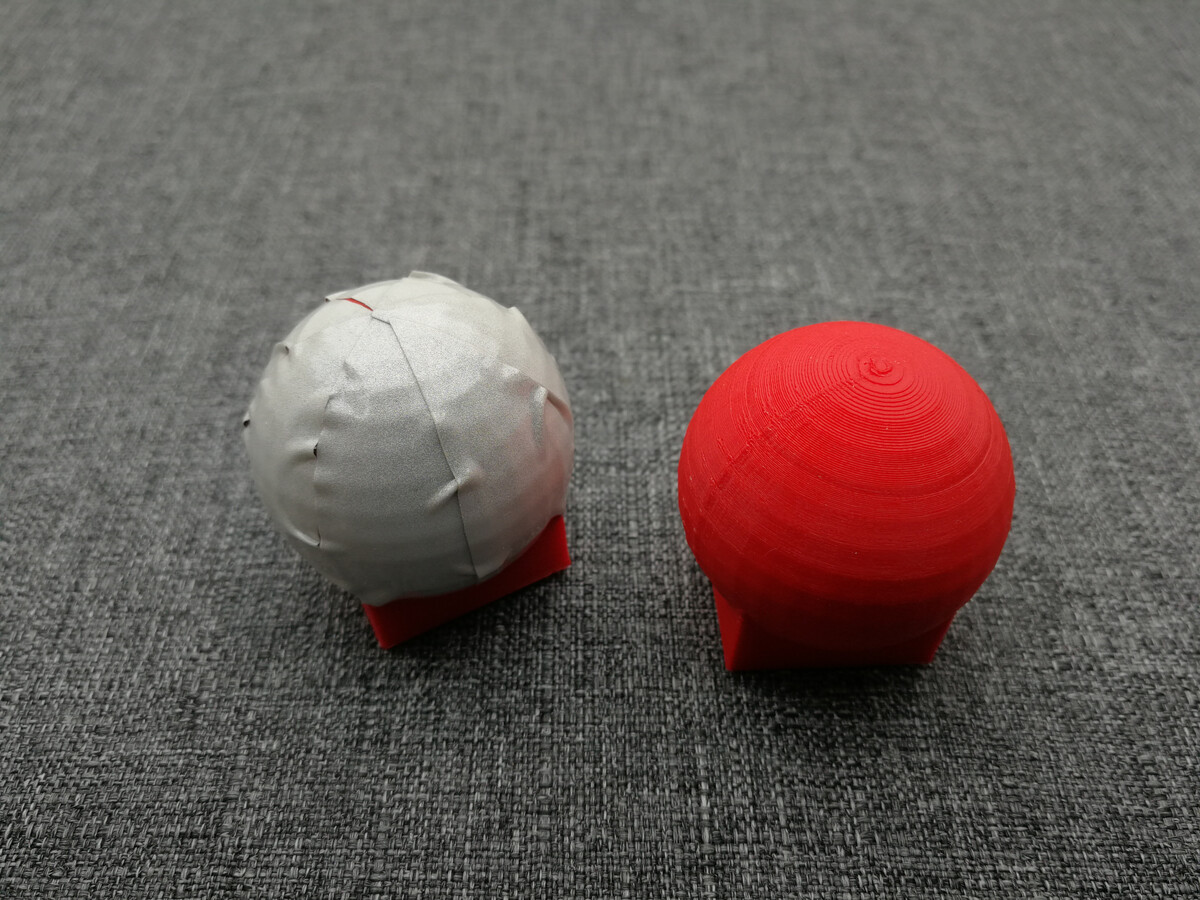3D printed markers, 30mm spheres with square base. Left marker is wrapped in cheap retroreflective tape.

One marker has been coated in reflective tape the other is simply the mildly reflective PLA print material. In a future post we'll look at the 3D model, cut out templates and different reflective materials. For now, let's look how a single frame from the camera looks: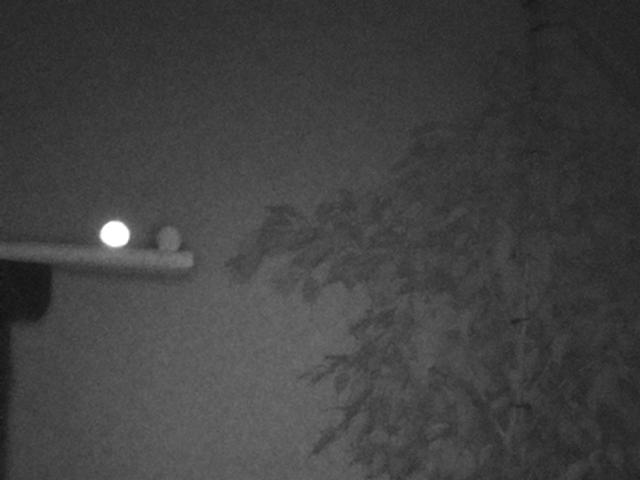Luminance image NoIR camera (mode 7) with 850nm filter and IR light source. Two markers are in the scene, the left is covered with reflective material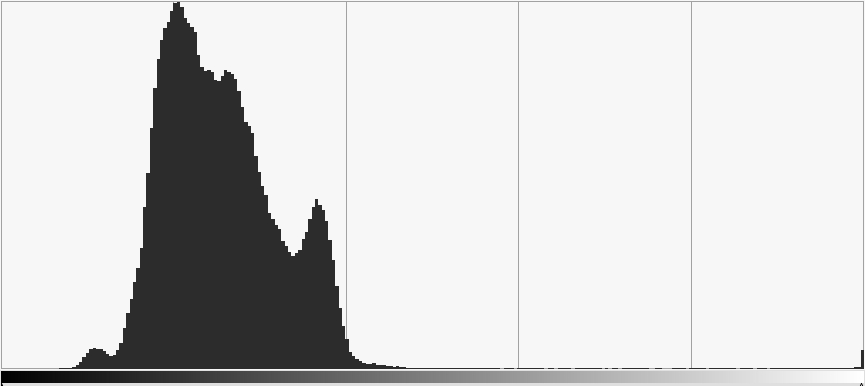Luminance frequency diagram of image, notice a small peak from reflective marker all the way on the right.

From the frequency diagram it is pretty clear that distinguishing the marker pixels from the image is exceptionally simple. A simple threshold value any where between 128 and 250 will filter only the marker: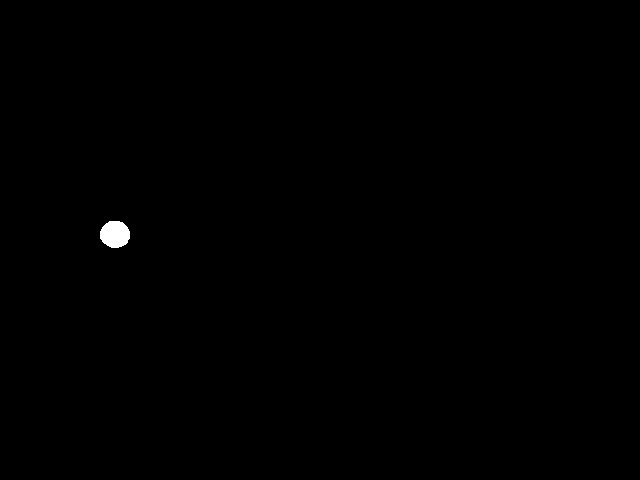Camera still 160 threshold. Luminance <160 -> black, >= 160 -> white.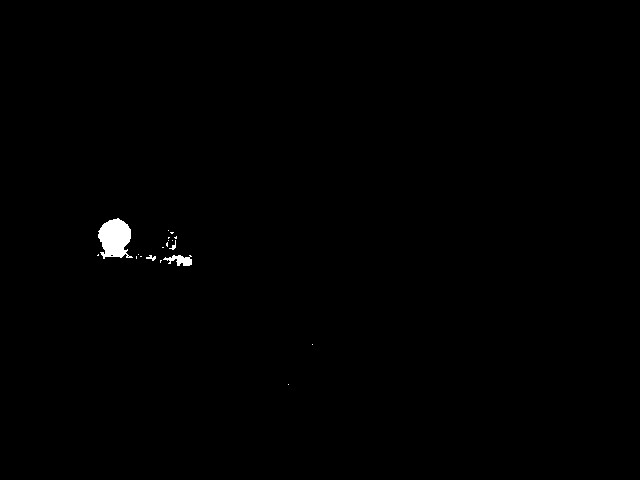Camera still 111 threshold. Luminance <111 -> black, >= 111 -> white.

As you can see, with a threshold of 160 we get a perfect binary image. Having such a perfect result with such a range of thresholds is superb. I promise you that image recognition is normally a lot harder than this! It shows the importance of using a IR light source and IR pass filter.

To increase robustness of further steps I'm going to murky the results of this step a bit. Say somehow a bit more noise came true, such as with a threshold of 111, how do we deal with this and how can we extract the marker center?

## Noise reduction

To get rid of the noise we can do a morphological opening. This will open up parts of the image that are small and of a different shape than our template.

Scikit-image morphology can do this operation for us.

It is quite a long operation though. On my fast laptop the operation with a disk of size 5 takes almost 30 of the total 40ms processing time:

Threshold 0.1ms
Opening 27.0ms
Connect 1.1ms
Center 13.6ms
Total 41.9ms

We might be able to speed this step up at a later time, for now let's use this and move on to extracting the position.

## Position extraction

The final step to get the marker position entails calculating the center of all remaining pixel blobs. This is a two step process:

1. Find all connected components, that is: all pixel blobs.
2. Compute center of these connected components.

Again we can use a library to do the heavy lifting for us.

The result is the extracted marker position: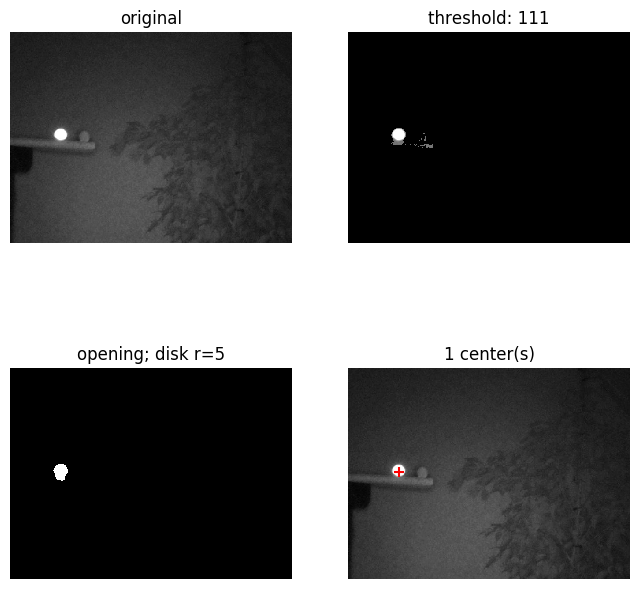Threshold, morphological opening and connected component centroid steps for single marker image.

## Code

All described steps are performed with the following experimental code:

``````"""
Detect markers in image and display results
"""
import time
import numpy as np

import matplotlib.pyplot as plt
from scipy import ndimage
from skimage import io
from skimage import morphology

def do_threshold(array, value):
return (array >= value) * array

def difference_ms(prev_t):
return round((time.time() - prev_t) * 1000, 1)

def main(path):

threshold = 160
opening_size = 5

t0 = time.time()
thresholded = do_threshold(original, threshold)  # very slow
print(f'Threshold:\t{difference_ms(t0)}ms')

t1 = time.time()
structure = morphology.disk(opening_size)
opened = ndimage.binary_opening(thresholded, structure)  # quite slow
structure = np.ones((3, 3), dtype=np.int)
print(f'Opening:\t\t{difference_ms(t1)}ms')

t2 = time.time()
labels, ncomponents = ndimage.label(opened)  # fast
print(f'Connect:\t\t{difference_ms(t2)}ms')

t3 = time.time()
centers = ndimage.measurements.center_of_mass(opened, labels, range(1, ncomponents + 1))  # fast
# Note, performing moment calculation on masked image may be faster (parallelizable).
print(f'Center:\t\t\t{difference_ms(t3)}ms')

total_ms = difference_ms(t0)
print(f'Total:\t\t\t{total_ms}ms')

print(f'ncomponents: {ncomponents}')
print(f'centers: {centers}')

#
# Plot
#
fig, axes = plt.subplots(2, 2, figsize=(8, 8), sharex=True, sharey=True)

axis = axes[0, 0]
axis.imshow(original, cmap=plt.cm.gray)
axis.set_title('original')
axis.axis('off')

axis = axes[0, 1]
axis.imshow(thresholded, cmap=plt.cm.gray)
axis.set_title(f'threshold: {threshold}')
axis.axis('off')

axis = axes[1, 0]
axis.imshow(opened, cmap=plt.cm.gray)
axis.set_title(f'opening; disk r={opening_size}')
axis.axis('off')

axis = axes[1, 1]
axis.imshow(original, cmap=plt.cm.gray)
axis.set_title(f'{ncomponents} center(s)')
axis.scatter(np.array(centers)[:, 1], np.array(centers)[:, 0], s=50, c='red', marker='+')
axis.axis('off')

# fig.suptitle(f'Total {total_ms}ms', fontsize=12)
# fig.tight_layout(rect=[0, 0.03, 1, 0.95])

plt.savefig('plot.png', bbox_inches='tight')
plt.show()

fig, axis = plt.subplots(1, 1, figsize=(8, 8), sharex=True, sharey=True)
axis.imshow(original, cmap=plt.cm.gray)
axis.scatter(np.array(centers)[:, 1], np.array(centers)[:, 0], s=50, c='red', marker='+')
axis.axis('off')
plt.savefig('result.png', bbox_inches='tight')

if __name__ == "__main__":
path = 'luminance_raw_with_IR_light.jpg'
path = 'more-marker-raw_IR_light.jpg'
main(path)
``````